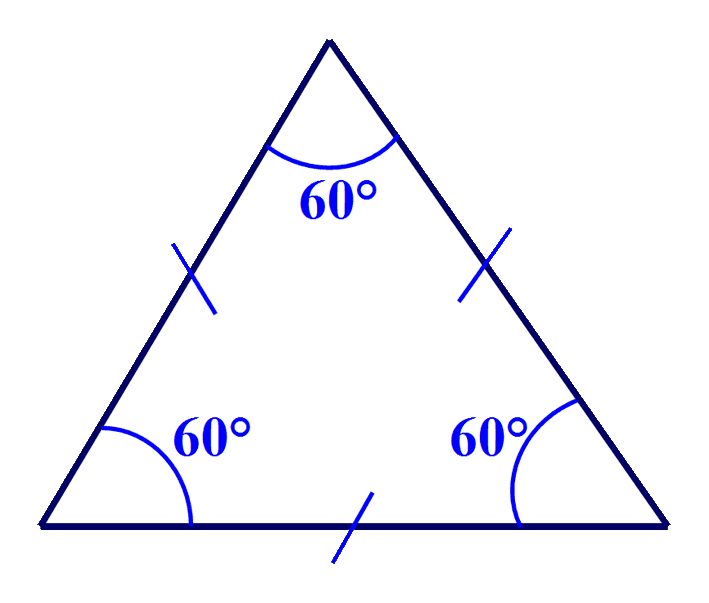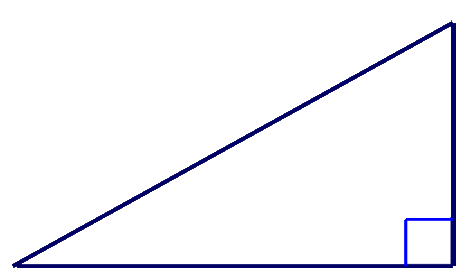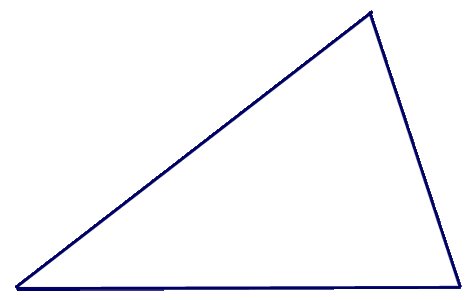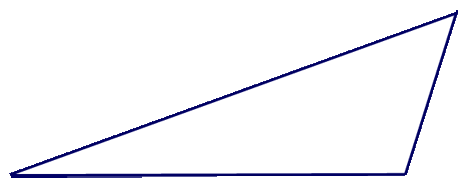# 2D Shapes - Triangles

### Triangles are 2 dimensional shapes which have three sides, three angles and three vertices. The three angles of a triangle always add up to 180°.

There are four types of triangle: Equilateral, Scalene, Isosceles and Right-Angled.

_________________________________________________________

# Equilateral Triangle

## 3 equal angles

The angles of a triangle always add up to 180°, therefore each angle of an equilateral triangle
is, 180 ÷ 3 = 60°# Isosceles Triangle

## 2 equal angles# Scalene Triangle

## No equal angles# Right-Angled Triangle

## One angle is a Right Angle (90°)# Acute-Angled Triangle

## 3 Acute angles# Obtuse-Angled Triangle

## 1 Obtuse angle_________________________________________________________